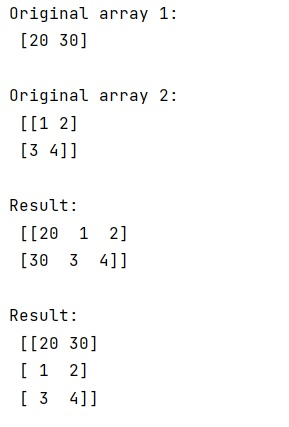# How to concatenate 2D arrays with 1D array in NumPy?

Learn, how to concatenate 2D arrays with 1D array in Python NumPy?
Submitted by Pranit Sharma, on March 06, 2023

## NumPy - Concatenate 2D arrays with 1D array

Suppose that we need to concatenate two arrays, one 1D array [7,8,4,2,7] and one 2D array of shape (2,3). We need to find a way so we can concatenate these arrays together while maintaining the dimensions.

To concatenate 2D arrays with 1D array in NumPy, we have different approaches, we can use ravel() to flatten the 2D array and then we can use concatenate so that the result would be a 1D array with all the elements of both the array together. Secondly, we can use the column_stack() method to stack the 1D array to the 2D array along the column and third we can use the row_stack() method to stack the 1D array to the 2D array along the rows.

Let us understand with the help of an example,

## Python code to concatenate 2D arrays with 1D array in NumPy

```# Import numpy
import numpy as np

# Creating arrays
arr1 = np.array([20, 30])
arr2 = np.array( [ [1,2],[3,4] ] )

# Display Original arrays
print("Original array 1:\n",arr1,"\n")
print("Original array 2:\n",arr2,"\n")

# using column stack
res = np.column_stack([arr1,arr2])

# Display result
print("Result:\n",res,"\n")

# using row stack
res = np.row_stack([arr1,arr2])

# Display result
print("Result:\n",res)
```

Output:Languages: » C » C++ » C++ STL » Java » Data Structure » C#.Net » Android » Kotlin » SQL
Web Technologies: » PHP » Python » JavaScript » CSS » Ajax » Node.js » Web programming/HTML
Solved programs: » C » C++ » DS » Java » C#
Aptitude que. & ans.: » C » C++ » Java » DBMS
Interview que. & ans.: » C » Embedded C » Java » SEO » HR
CS Subjects: » CS Basics » O.S. » Networks » DBMS » Embedded Systems » Cloud Computing
» Machine learning » CS Organizations » Linux » DOS
More: » Articles » Puzzles » News/Updates